Best daily deals

# How to sum and subtract in Google Sheets

By
May 11, 2023

Did you know that your spreadsheet can double as a calculator? Google Sheets has various functions that do the math for you, which is especially helpful when handling large data sets. As with learning anything, it’s best to start with the basics. Here’s how to sum and subtract in Google Sheets.

To sum and subtract in Google Sheets, use the formula =SUM(x:y) or =MINUS(x,y) in the desired cells and input the relevant values. Press Enter to get the results.

KEY SECTIONS

## How to sum in Google Sheets

Adding up columns or rows of numbers is familiar to most spreadsheet programs. Google Sheets includes a built-in function named SUM to add the total value. There are two easy ways to utilize the SUM function.

The first is by inserting the function. To do so, click the cell you wish to insert the function, click Insert, Function, and select SUM. You’ve now added a SUM function but still have to input values.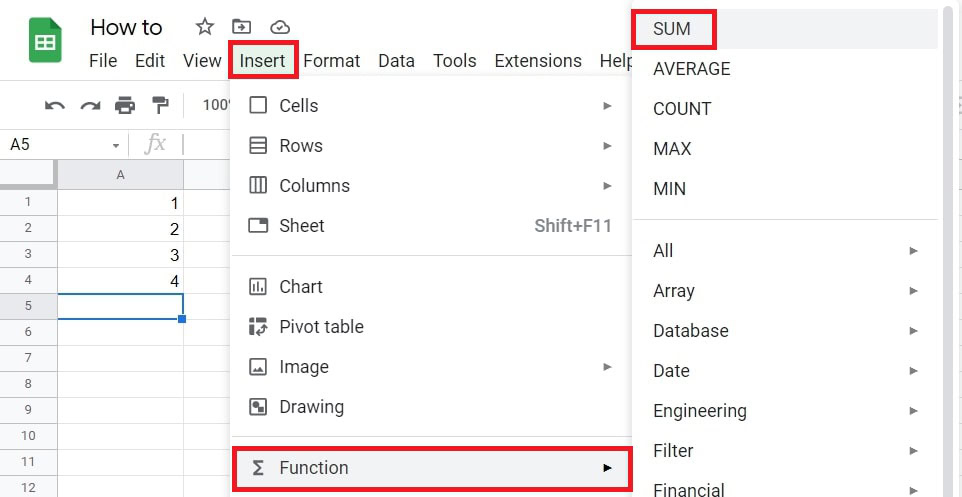In this example, we will add the numbers in column A. To input the values into the SUM function, you can click between the brackets of the SUM function and click and drag over the cells in column A. Alternatively, you can input the range of cells you want to total in the top formula bar beside f(x). In this case, the range is A1:A4.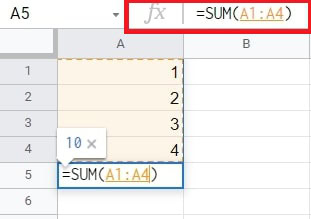You will notice that the function will preview the result in a small pop-up window, in this case, 10. When ready to sum, hit the Enter key, and the function result will appear in the cell.

Another way to use the SUM function is by writing the formula. To do so, type =SUM into the cell. As you can see in this example, because of where I have written the formula, the first suggestion for the input values is the range of column A.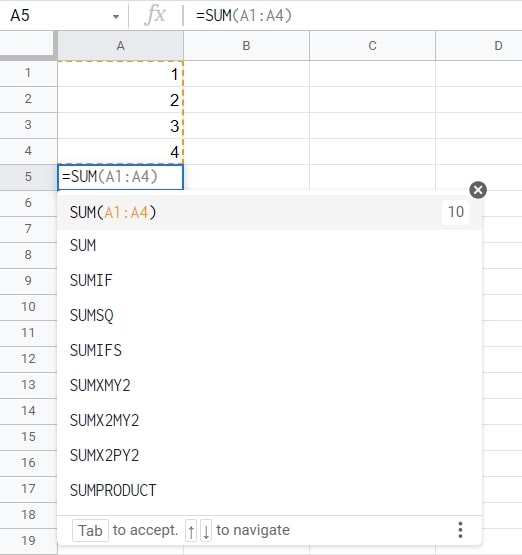As indicated, press Tab to accept the suggested values or type the desired values manually if they do not appear.

## How to subtract in Google Sheets

As with summing, subtracting can be done in two easy ways. For this example, we’ll subtract each expenditure value (column B) from each income value (column A) and place the resulting balance in column C.

First, type the = sign into the cell beside the row you want to subtract. This will cause Google Sheets to suggest the numbers to the left as input values, which you can select by pressing Tab. Otherwise, you can input any cell manually with a minus sign () between them.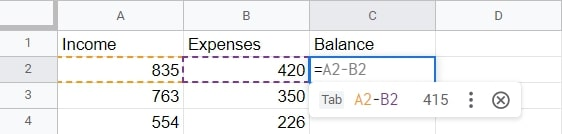Next, the difference is displayed in cell C2, and you’ll notice a small blue square in the bottom right corner. This is called the Fill Handle, and it’s pretty handy. You can click and drag the fill handle down the rest of the column to quickly subtract the remaining values.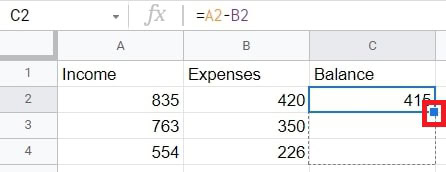Then, release the left-click on your mouse, and the differences in each row’s values will be displayed down the column. This saves you time from writing the function over and over.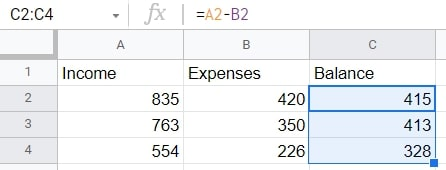Another way of subtracting in Google Sheets is by using the MINUS function. You can find the function from the Insert tab or type it in the cell as =MINUS.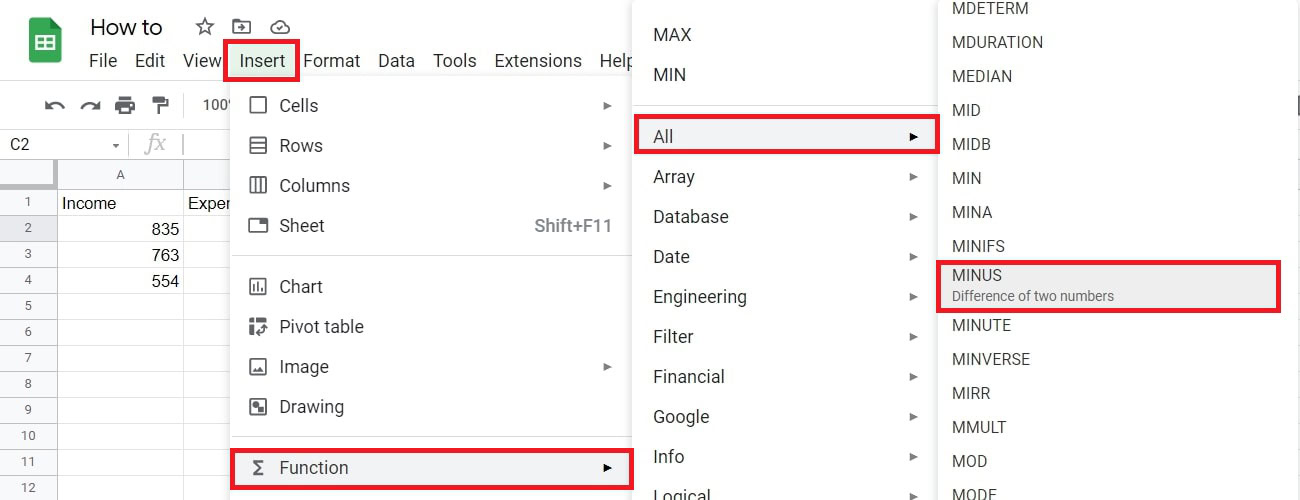Next, the function may suggest using the values of the row, which you can accept by pressing Tab.

Otherwise, you can type the cell containing the number you want to subtract from, followed by a comma, and the cell containing the number you wish to deduct. They can be typed into the function’s brackets or above the formula bar. In this case, we will use (A2, B2).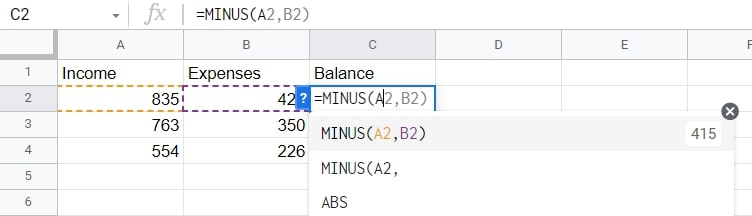As with the previous example, you can click and drag the Fill Handle to apply the function to the rest of the rows.

## How to solve errors when adding and subtracting in Google Sheets

Whenever you enter a formula into a cell, and the spreadsheet software cannot understand what you want it to do, we call that a parse error. The two common causes for this error are a typo in your formula or unclear order of operations. For example, typing =+ instead of =, forgetting to put quotation marks around text values, or leaving out parentheses are all things we can easily miss.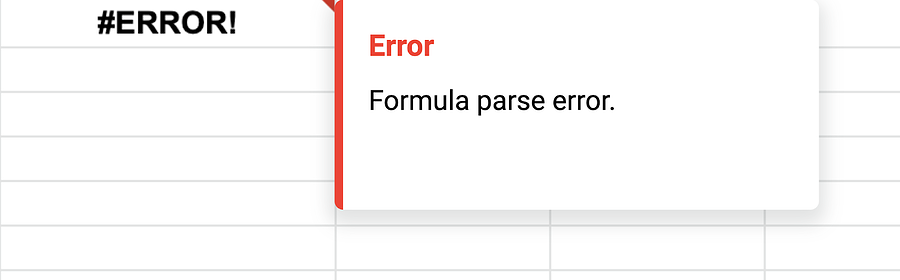Here’s a list of errors and why your formulas might not work correctly.

• #N/A Error: One of the most common errors is when a formula can’t find what it’s looking for. Check your formula inputs and make sure they’re correct.
• #DIV/0 Error: This happens when you try to divide a number by zero. Don’t do that.
• #REF! Error: You will get this error message when a formula contains an invalid cell reference; for example, if you have a formula that references cells B15:B25 and delete row 24, the formula will return the #REF! Error because it no longer has a valid reference.
• #VALUE Error: This error occurs when a formula contains an invalid value. For example, if you have a formula that adds two cells and one of the cells contains text instead of a number, you’ll get this error.
• #NAME Error: This error occurs when a formula contains an invalid name. For example, if you have a named range called “Expenses” and you accidentally type “s” at the end of your formula, you’ll get the #NAME? error
• #NUM Error: The #NUM! error occurs when a formula contains an invalid number. This can happen when a formula is applied to two or more cells, and the result is too large to display.

Remember to double-check your formula inputs and ensure you’re using the correct operators.

### FAQs

Insert the MULTIPLY function into the relevant cell, or type =MULTIPLY(x,y) into the cell, then write the desired input values between the brackets.

You can use the fill handle feature to subtract multiple cells in Google Sheets. After applying the MINUS function to one cell, you can click and drag its bottom right corner down or across the row or columns of cells you want to apply the function to derive results quickly.